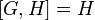# A5 in S5

This article is about a particular subgroup in a group, up to equivalence of subgroups (i.e., an isomorphism of groups that induces the corresponding isomorphism of subgroups). The subgroup is (up to isomorphism) alternating group:A5 and the group is (up to isomorphism) symmetric group:S5 (see subgroup structure of symmetric group:S5).
The subgroup is a normal subgroup and the quotient group is isomorphic to cyclic group:Z2.
VIEW: Group-subgroup pairs with the same subgroup part | Group-subgroup pairs with the same group part| Group-subgroup pairs with the same quotient part | All pages on particular subgroups in groups

## Definition

We define$G$ as symmetric group:S5 -- for concreteness, the symmetric group on the set$\{ 1,2,3,4,5 \}$.$H$ is alternating group:A5 -- the subgroup of$G$ comprising even permutations. A criterion for a permutation to be even, based on cycle decomposition, is that the number of cycles of even length should be even.

## Arithmetic functions

Function Value Explanation
order of group 120 Order is$5! = 5 \cdot 4 \cdot 3 \cdot 2 \cdot 1 = 120$
order of subgroup 60 Order is$5!/2 = 120/2 = 60$
index of subgroup 2 For$n \ge 2$, the alternating group has index two in the symmetric group, since it has two cosets: itself (the even permutations) and the odd permutations.

## Subgroup-defining functions

Subgroup-defining function Meaning in general Why it takes this value GAP verification (set G := SymmetricGroup(5); H := AlternatingGroup(5);)
derived subgroup (also called commutator subgroup) subgroup generated by commutators Since the quotient is abelian, it contains the derived subgroup. Further,$H$ is simple non-abelian, so the derived subgroup cannot be smaller. H = DerivedSubgroup(G); using DerivedSubgroup
hypocenter stable member of lower central series (transfinite if necessary)$H$ is the derived subgroup, and$[G,H] = H$
perfect core stable member of derived series (transfinite if necessary); alternatively, join of all perfect subgroups$H$ is perfect, and the only bigger subgroup is$G$, which is not perfect because$[G,G] = H$

## GAP implementation

We can define the group and subgroup naturally, using the SymmetricGroup and AlternatingGroup functions:

G := SymmetricGroup(5); H := AlternatingGroup(5);